Get instant live expert help with Excel or Google Sheets“My Excelchat expert helped me in less than 20 minutes, saving me what would have been 5 hours of work!”

#### Post your problem and you’ll get expert help in seconds.

Your message must be at least 40 characters
Our professional experts are available now. Your privacy is guaranteed.

# Get first Monday before any date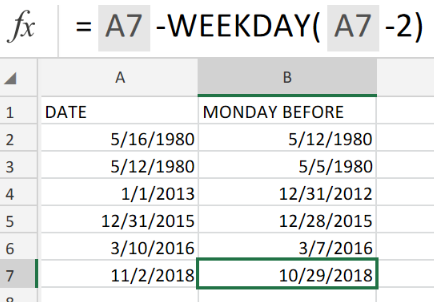Figure 1. Get First Monday Before Any Date in Excel.

Let’s assume that we are required to find the first Monday occurring before a specific date in Excel, we will have to make use of the WEEKDAY function.

## Generic Formula

`=date- WEEKDAY (date - 2)`

The WEEKDAY formula will help us to look back in time to locate the nearest Monday before a specific date.

## How to use the Excel WORKDAY Function.

To use the WORKDAY Function in Excel, follow these 3 simple steps;

1. List out the dates we want Excel to reference in a labeled column of our worksheet.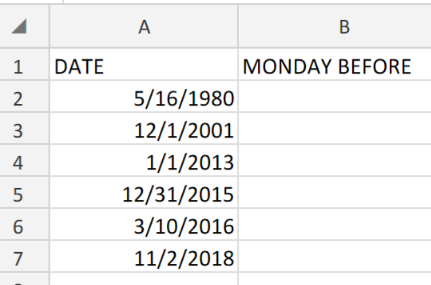Figure 2. Get First Monday Before Any Date in Excel.

Our purpose here is to determine the first Monday occuring before the dates in column A of our worksheet.

1. In the example above, the WORKDAY formula which we are going to enter into cell B2, is as follows;

`=A2-WEEKDAY(A2-2)`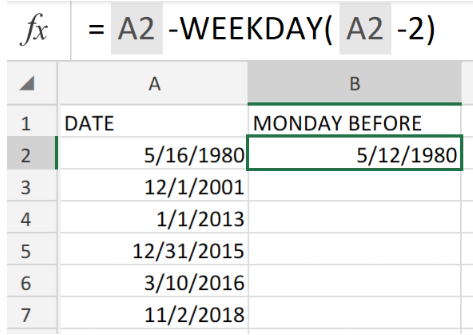Figure 3. Get First Monday Before Any Date in Excel.

The Excel WORKDAY Function automatically returned 5)12/1980 as the first Monday occuring before the date in cell A2.

1. Edit the WORKDAY formula in cell B2 and copy into the other cells in the MONDAY BEFORE column to achieve the required results.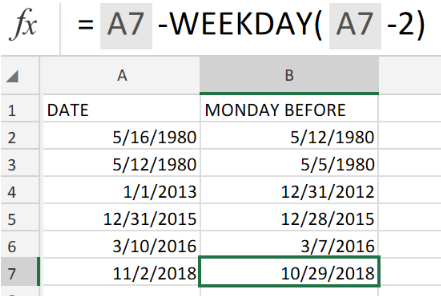Figure 4. Get First Monday Before Any Date in Excel.

The Excel WORKDAY Function is based on Excel’s default scheme, where Monday is recognized as 2 in a week beginning on Sunday.

Excel calculates roll back by obtaining the actual value of the weekday of the date, two  days ahead of our specified date, and then it subtracts this value from our date.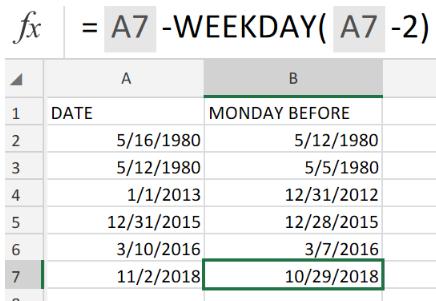Figure 5. Final Result.

## Instant Connection to an Expert through our Excelchat Service:

Our live Excelchat Service is here for you. We have Excel Experts available 24/7 to answer any Excel questions you may have. Guaranteed connection within 30 seconds and a customized solution for you within 20 minutes.

### Did this post not answer your question? Get a solution from connecting with the expert.Another blog reader asked this question today on Excelchat:
Solution examplesI need to create a formula where column D becomes the date in column C + 5 business days when I enter a date into column C
Solved by X. Q. in 14 minsneed help with the different sheets and formula IF(A4=B4,0,NETWORKDAYS(A4+1,B4,\$E\$9:\$E\$42) +0.5*(INT((+B4-A4+1)/7)+IF(WEEKDAY(A4+1)+MOD (B4-A4-1,7)>=7,1,0)))
Solved by O. D. in 11 minsi have this formula currently which works but not if I want it for every day - any ideas ?? (NETWORKDAYS.INTL(L1362,M1362,11,BH!A:A)-1)*("17:30"-"8:30")+IF(NETWORKDAYS.INTL(M1362,M1362,11,BH!A:A),MEDIAN(MOD(M1362,1),"8:30","17:30"),"17:30")-MEDIAN(NETWORKDAYS.INTL(L1362,L1362,11,BH!A:A)*MOD(L1362,1),"8:30","17:30")
Solved by A. D. in 28 mins## Subscribe to Excelchat.coAnother blog reader asked this question today on Excelchat: